Examples

Chapter 3 Class 10 Pair of Linear Equations in Two Variables
Serial order wise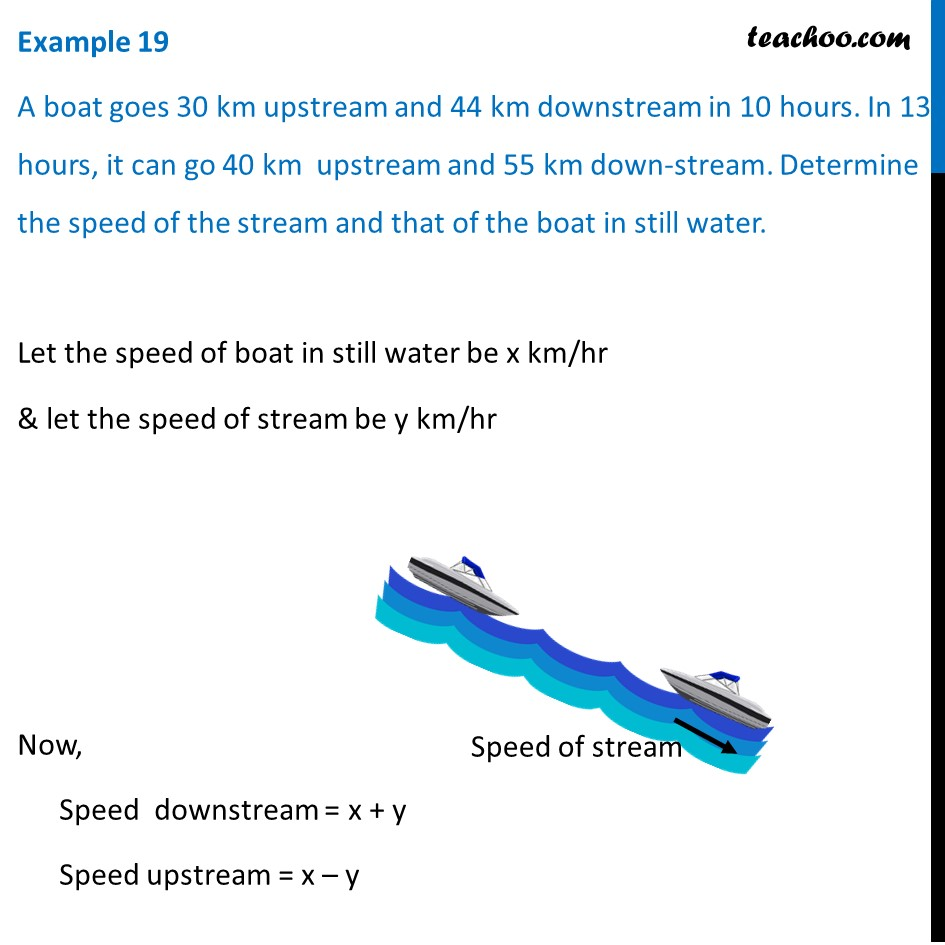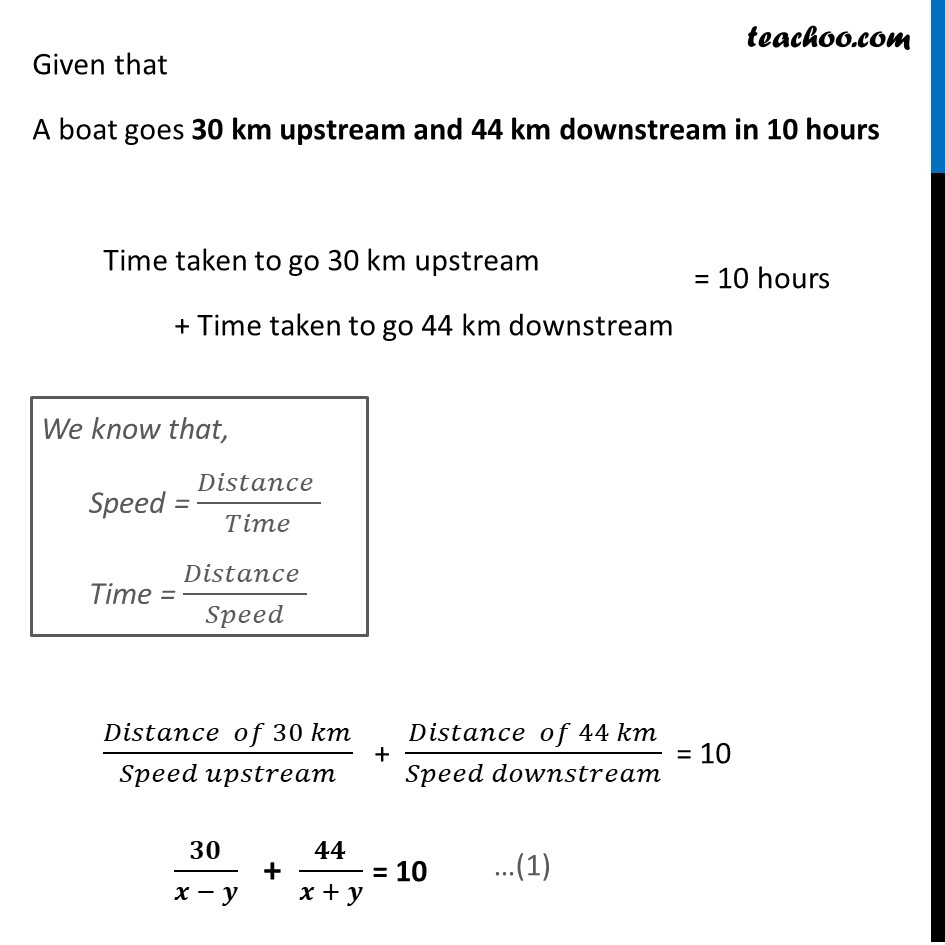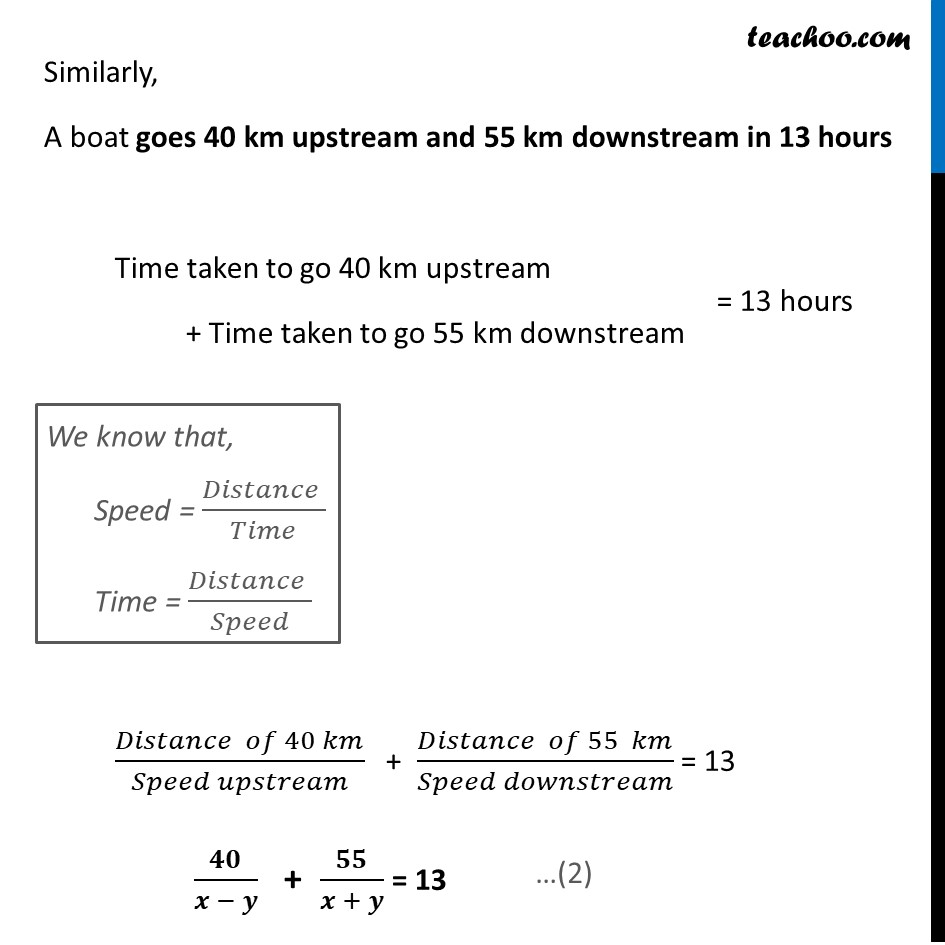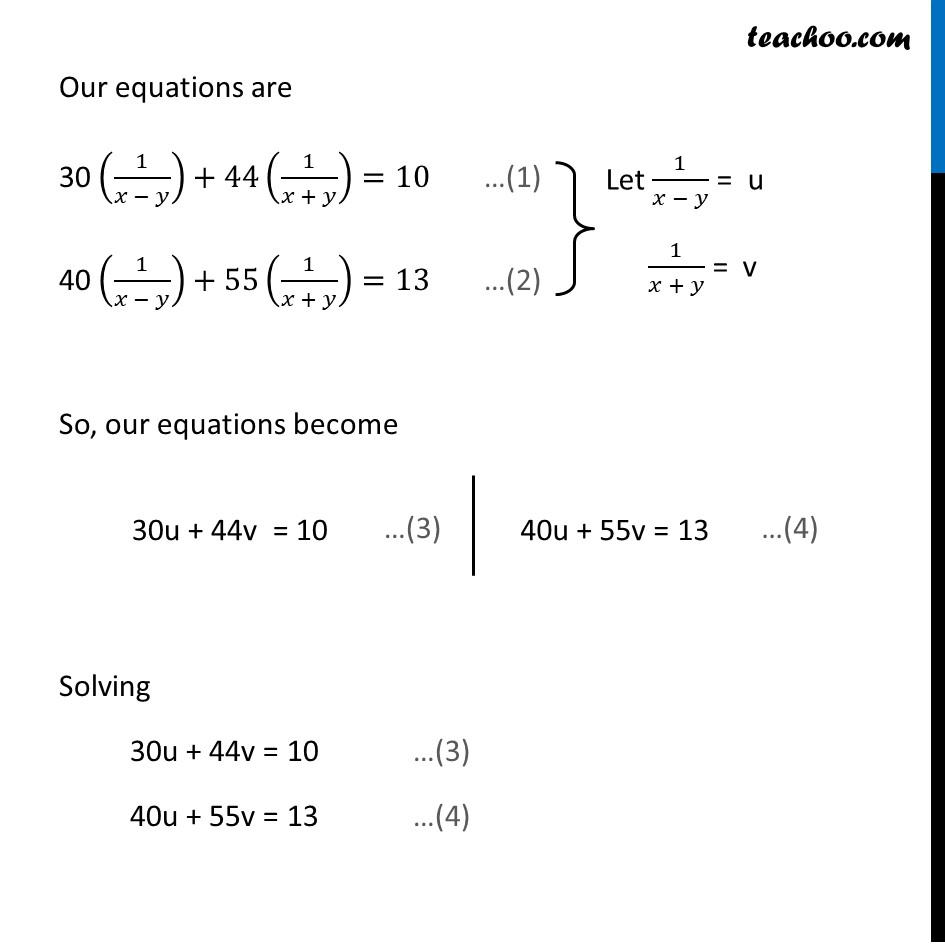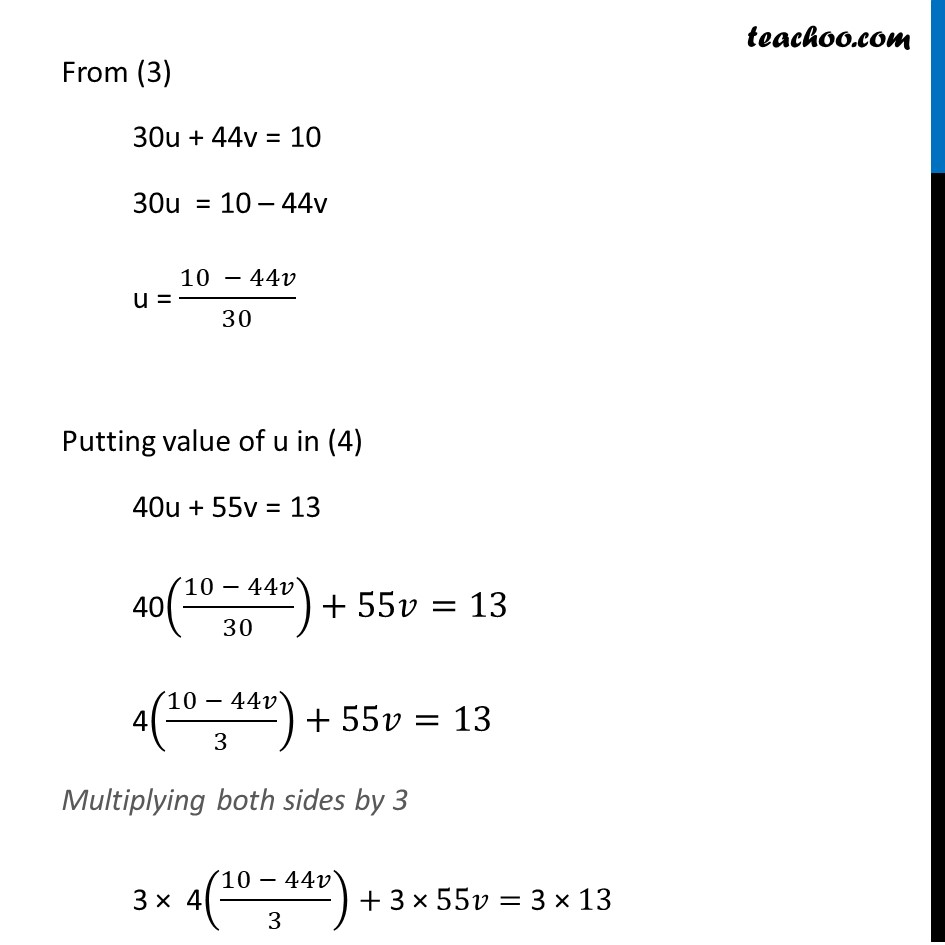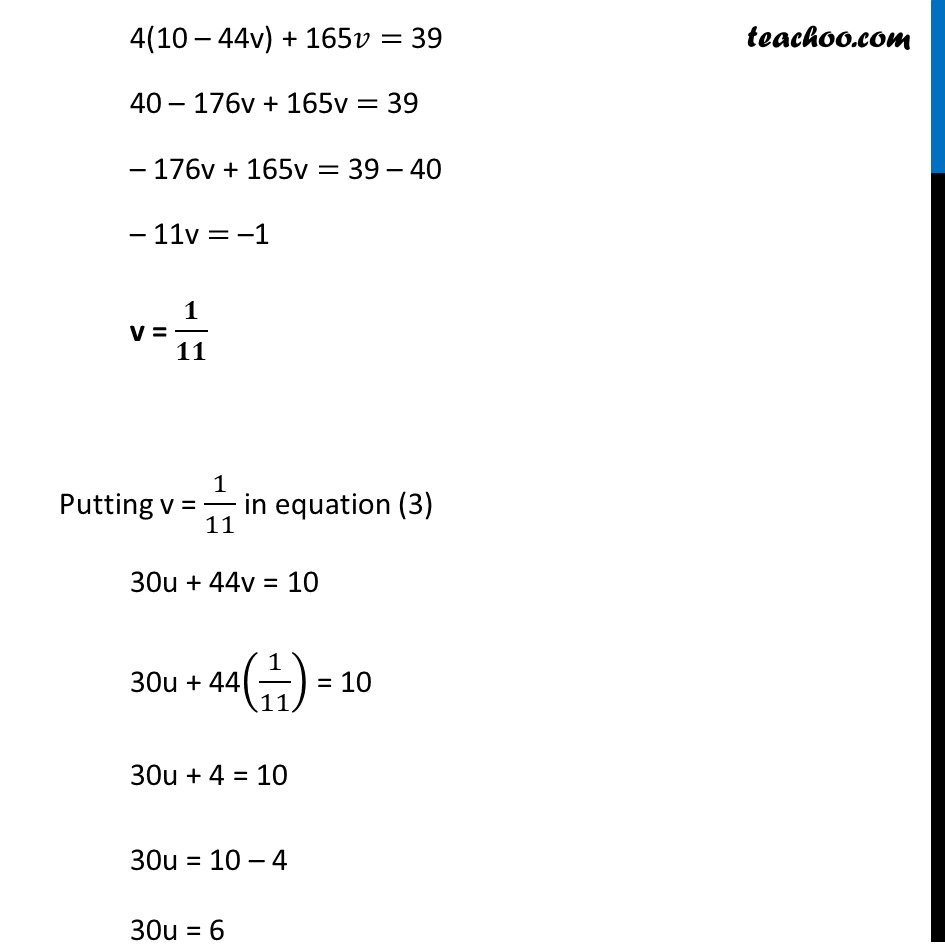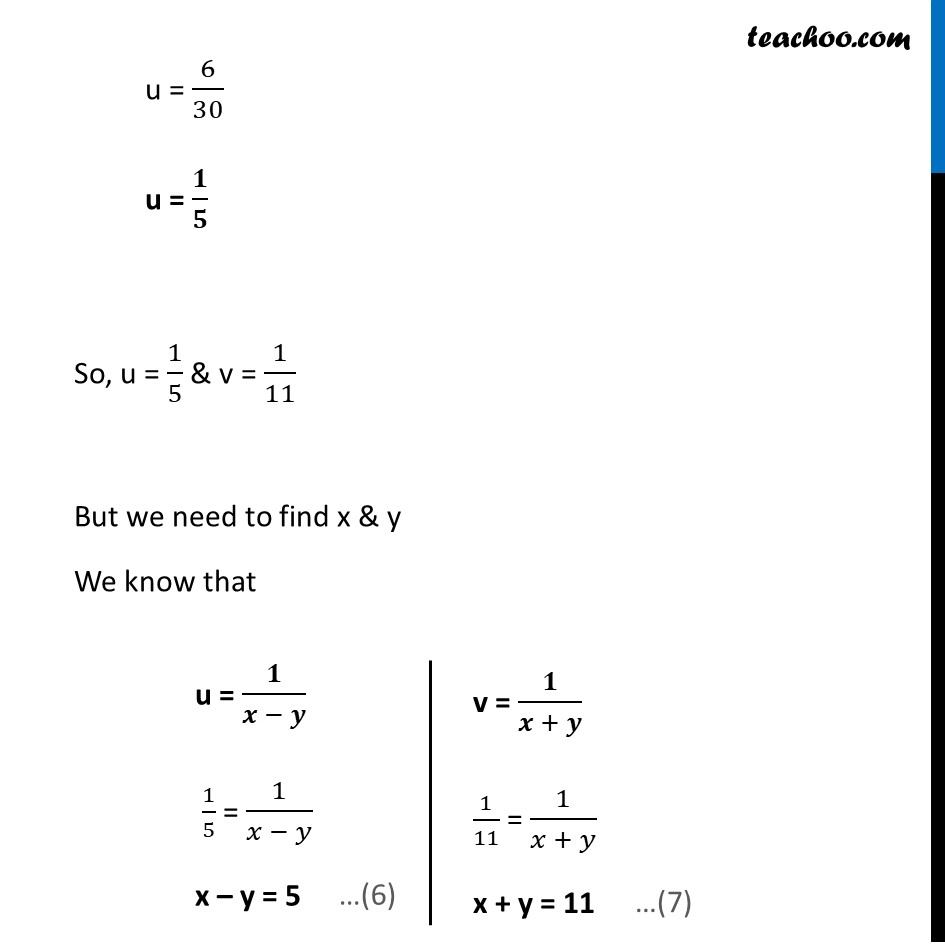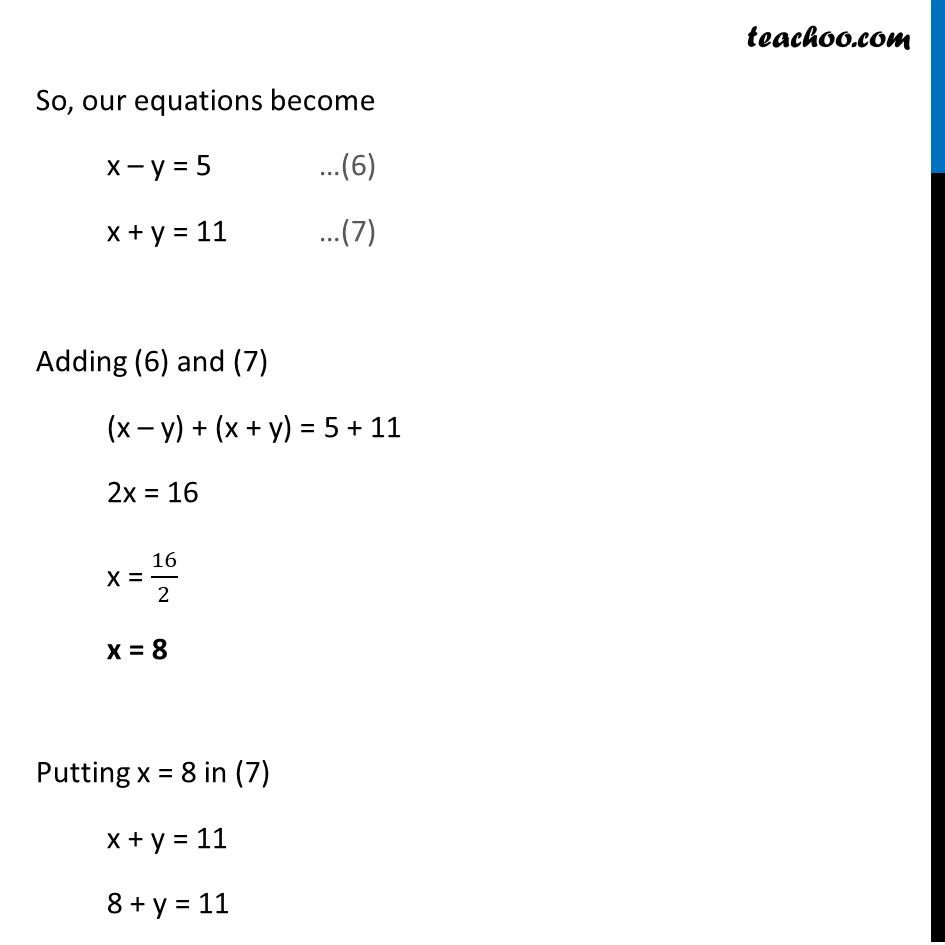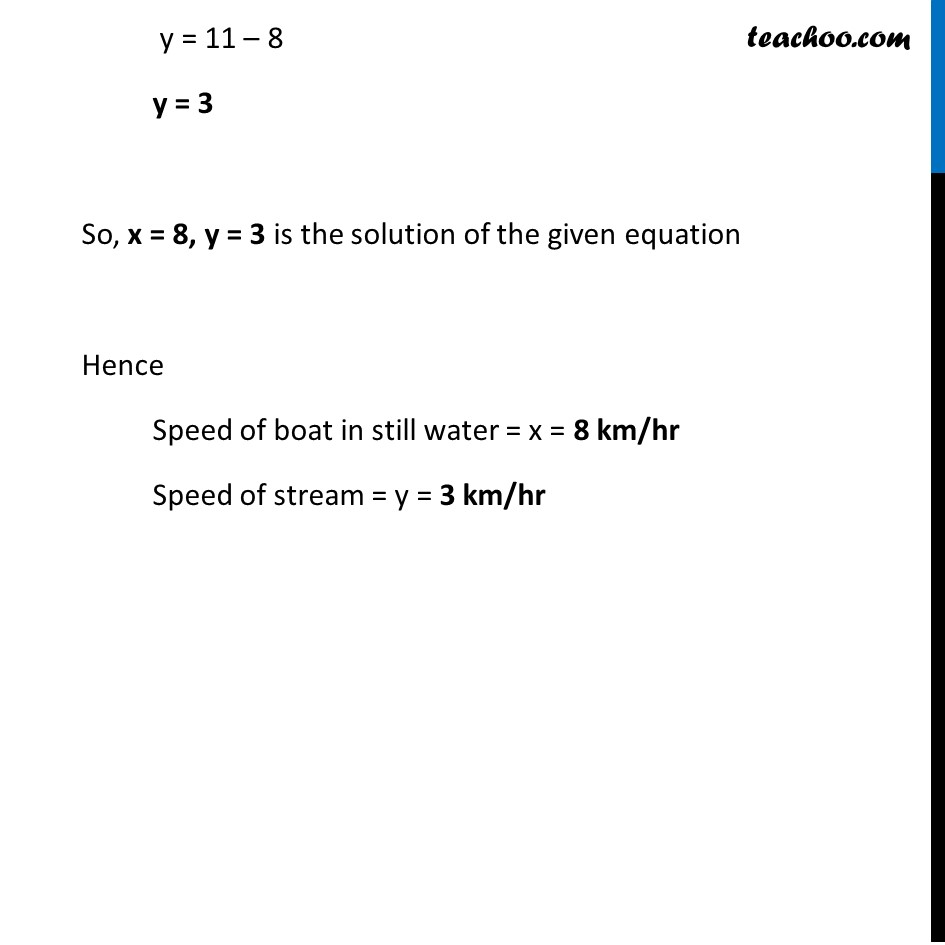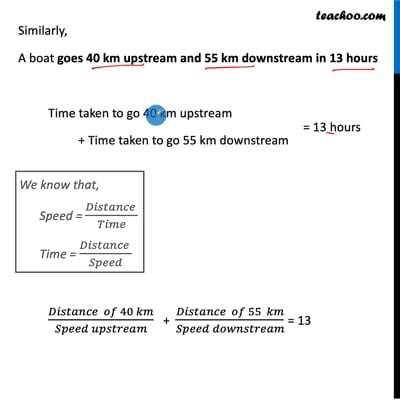This video is only available for Teachoo black users

Solve all your doubts with Teachoo Black (new monthly pack available now!)

### Transcript

Example 19 A boat goes 30 km upstream and 44 km downstream in 10 hours. In 13 hours, it can go 40 km upstream and 55 km down-stream. Determine the speed of the stream and that of the boat in still water. Let the speed of boat in still water be x km/hr & let the speed of stream be y km/hr Now, Speed downstream = x + y Speed upstream = x – y Given that A boat goes 30 km upstream and 44 km downstream in 10 hours Time taken to go 30 km upstream + Time taken to go 44 km downstream (𝐷𝑖𝑠𝑡𝑎𝑛𝑐𝑒 𝑜𝑓 30 𝑘𝑚)/(𝑆𝑝𝑒𝑒𝑑 𝑢𝑝𝑠𝑡𝑟𝑒𝑎𝑚) + (𝐷𝑖𝑠𝑡𝑎𝑛𝑐𝑒 𝑜𝑓 44 𝑘𝑚)/(𝑆𝑝𝑒𝑒𝑑 𝑑𝑜𝑤𝑛𝑠𝑡𝑟𝑒𝑎𝑚) = 10 𝟑𝟎/(𝒙 − 𝒚) + 𝟒𝟒/(𝒙 + 𝒚) = 10 Similarly, A boat goes 40 km upstream and 55 km downstream in 13 hours Time taken to go 40 km upstream + Time taken to go 55 km downstream (𝐷𝑖𝑠𝑡𝑎𝑛𝑐𝑒 𝑜𝑓 40 𝑘𝑚)/(𝑆𝑝𝑒𝑒𝑑 𝑢𝑝𝑠𝑡𝑟𝑒𝑎𝑚) + (𝐷𝑖𝑠𝑡𝑎𝑛𝑐𝑒 𝑜𝑓 55 𝑘𝑚)/(𝑆𝑝𝑒𝑒𝑑 𝑑𝑜𝑤𝑛𝑠𝑡𝑟𝑒𝑎𝑚) = 13 𝟒𝟎/(𝒙 − 𝒚) + 𝟓𝟓/(𝒙 + 𝒚) = 13 Our equations are 30 (1/(𝑥 − 𝑦))+44(1/(𝑥 + 𝑦))=10 …(1) 40 (1/(𝑥 − 𝑦))+55(1/(𝑥 + 𝑦))=13 …(2) So, our equations become 30u + 44v = 10 40u + 55v = 13 Solving 30u + 44v = 10 …(3) 40u + 55v = 13 …(4) From (3) 30u + 44v = 10 30u = 10 – 44v u = (10 − 44𝑣)/30 Putting value of u in (4) 40u + 55v = 13 40((10 − 44𝑣)/30)+55𝑣=13 4((10 − 44𝑣)/3)+55𝑣=13 Multiplying both sides by 3 3 × 4((10 − 44𝑣)/3)+"3 ×" 55𝑣="3 ×" 13 4(10 – 44v) + 165𝑣= 39 40 – 176v + 165v = 39 – 176v + 165v = 39 – 40 – 11v = –1 v = 𝟏/𝟏𝟏 Putting v = 1/11 in equation (3) 30u + 44v = 10 30u + 44(1/11) = 10 30u + 4 = 10 30u = 10 – 4 30u = 6 u = 6/30 u = 𝟏/𝟓 So, u = 1/5 & v = 1/11 But we need to find x & y We know that u = 𝟏/(𝒙 − 𝒚) 1/5 = 1/(𝑥 − 𝑦) x – y = 5 v = 𝟏/(𝒙 + 𝒚) 1/11 = 1/(𝑥 + 𝑦) x + y = 11 So, our equations become x – y = 5 …(6) x + y = 11 …(7) Adding (6) and (7) (x – y) + (x + y) = 5 + 11 2x = 16 x = 16/2 x = 8 Putting x = 8 in (7) x + y = 11 8 + y = 11 y = 11 – 8 y = 3 So, x = 8, y = 3 is the solution of the given equation Hence Speed of boat in still water = x = 8 km/hr Speed of stream = y = 3 km/hr